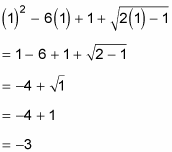##### Pre-Calculus Workbook For Dummies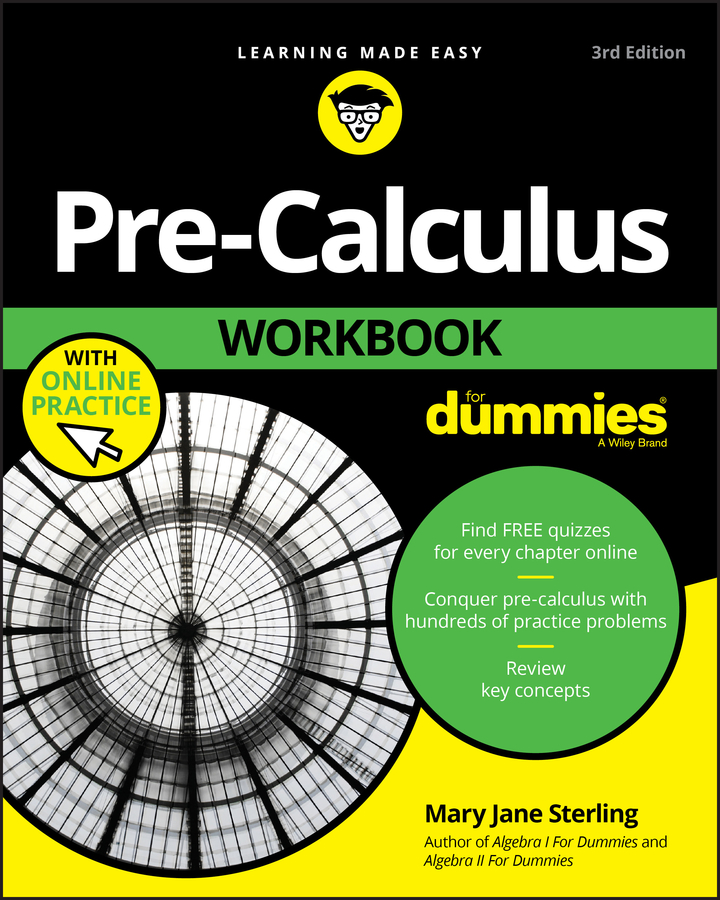In your pre-calculus class, you may be asked to operate with two or more functions. Operating with functions can involve addition, subtraction, multiplication, or division.

## Adding and subtracting two or more functions

When asked to add functions, you simply combine like terms, if the functions have any. For example, say you have three functions,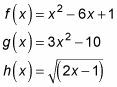Now let’s say that (f + g)(x) is asking you to add the f(x) and the g(x) functions: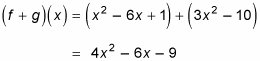The x2 and 3x2 add to 4x2; –6x remains because it has no like terms; 1 and –10 add to –9.

But what do you do if you’re asked to add (g + h)(x)? You get the following equation: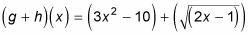You have no like terms to add, so you can’t simplify the answer any further. You’re done!

When asked to subtract functions, you distribute the negative sign throughout the second function, using the distributive property, and then treat the process like an addition problem: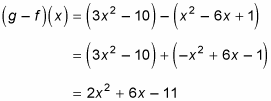## Multiplying and dividing two or more functions

Multiplying and dividing functions is a similar concept to adding and subtracting them. When multiplying functions, you use the distributive property over and over and then add the like terms to simplify. Dividing functions is trickier, however.

You’ll tackle multiplication first and save the trickster division for last. Here’s the setup for multiplying f(x) and g(x):

(fg)(x) = (x2 – 6x + 1)(3x2 – 10)

Follow these steps to multiply these functions:

1. Distribute each term of the polynomial on the left to each term of the polynomial on the right.

You start with x2(3x2) + x2(–10) + –6x(3x2) + –6x(–10) + 1(3x2) + 1(–10).

You end up with 3x4 – 10x2 – 18x3 + 60x + 3x2 – 10.

2. Combine like terms to get the final answer to the multiplication.

This simple step gives you 3x4 – 18x3 – 7x2 + 60x – 10.

Operations that call for division of functions may involve factoring to cancel out terms and simplify the fraction. If you’re asked to divide g(x) by f(x), though, you write the following equation: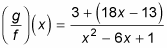Because neither the denominator nor the numerator factor, the new, combined function is simplified and you’re done.

You may be asked to find a specific value of a combined function. For example, (f + h)(1) asks you to put the value of 1 into the combined function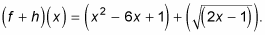When you plug in 1, you get